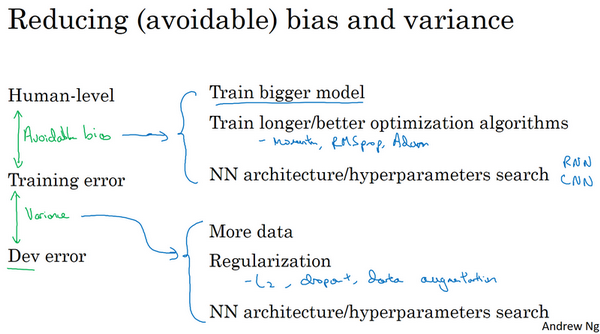# 第三门课 结构化机器学习项目（Structuring Machine Learning Projects）

## 第一周 机器学习（ML）策略（1）（ML strategy（1））

### 1.1 为什么是ML策略？（Why ML Strategy?）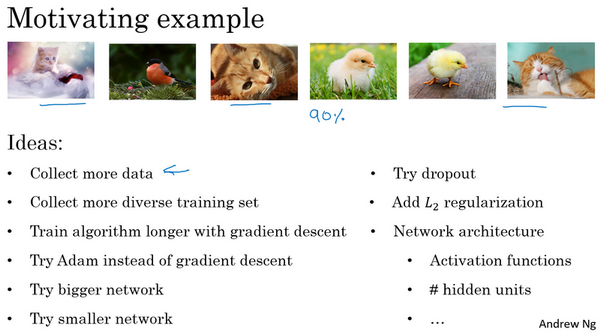### 1.2 正交化（Orthogonalization）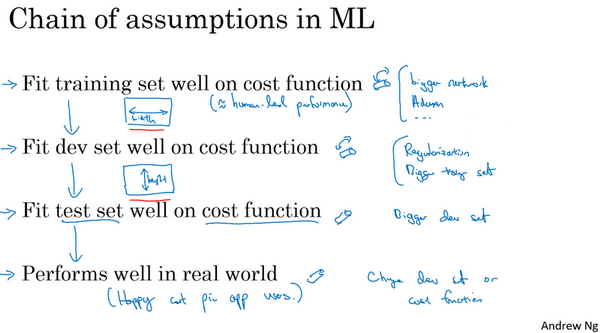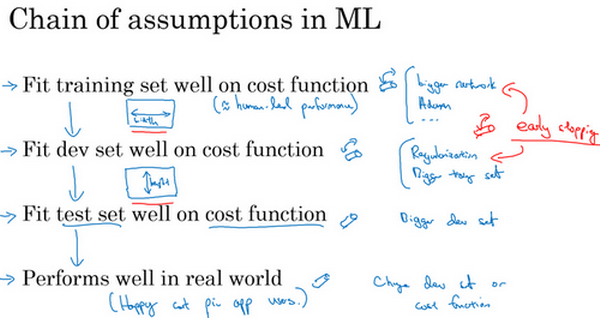### 1.3 单一数字评估指标（Single number evaluation metric）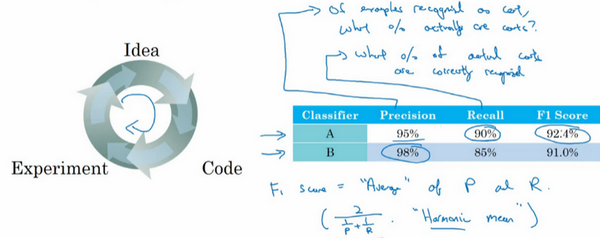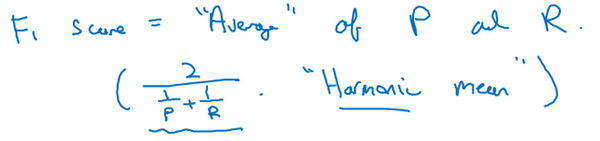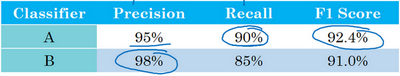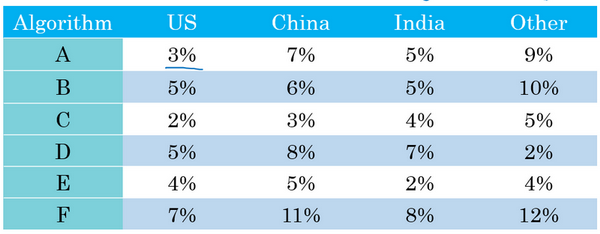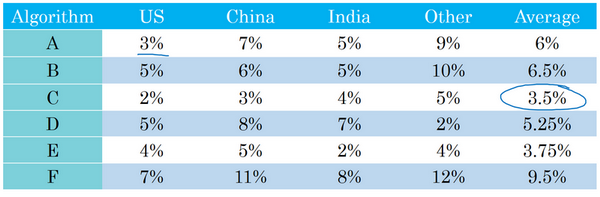### 1.4 满足和优化指标（Satisficing and optimizing metrics）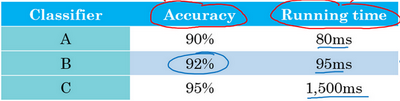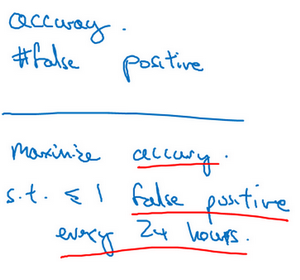### 1.5 训练/开发/测试集划分（Train/dev/test distributions）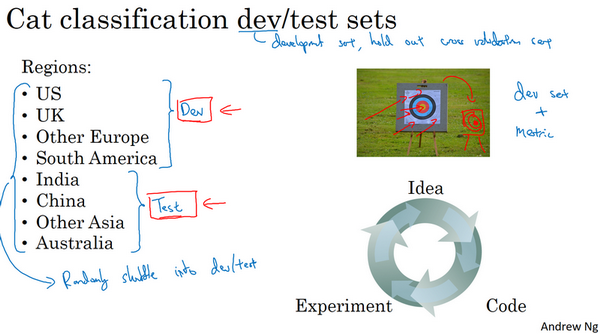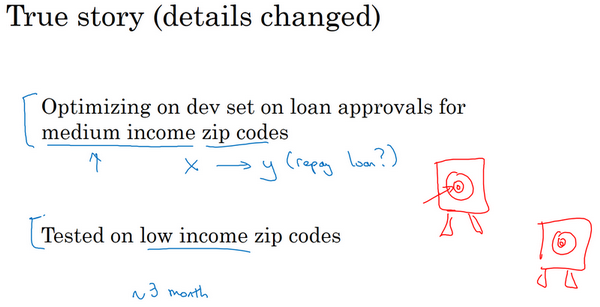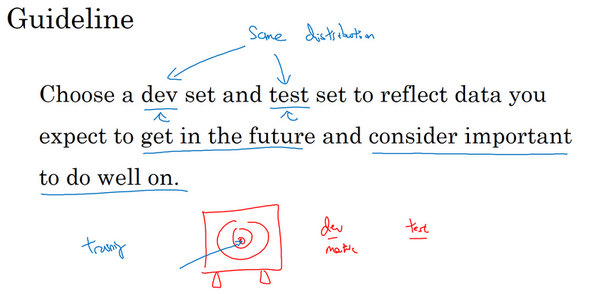### 1.6 开发集和测试集的大小（Size of dev and test sets）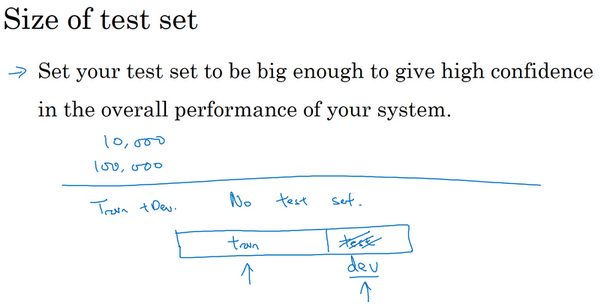### 1.7 什么时候该改变开发/测试集和指标？（When to change dev/test sets and metrics）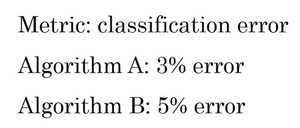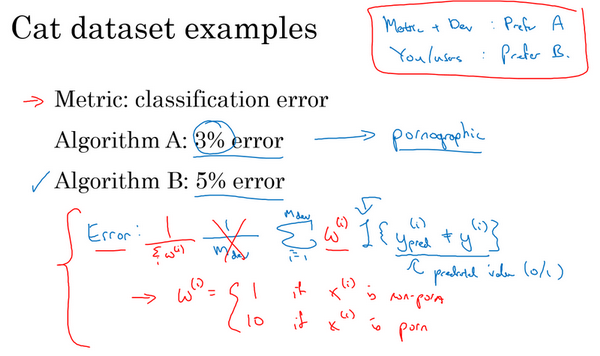$Error = \frac{1}{m_{{dev}}}\sum_{i = 1}^{m_{{dev}}}{I\{ y_{{pred}}^{(i)} \neq y^{(i)}\}}$

$m_{{dev}}$是你的开发集例子数，用$y_{{pred}}^{(i)}$表示预测值，其值为0或1，$I$这符号表示一个函数，统计出里面这个表达式为真的样本数，所以这个公式就统计了分类错误的样本。这个评估指标的问题在于，它对色情图片和非色情图片一视同仁，但你其实真的希望你的分类器不会错误标记色情图像。比如说把一张色情图片分类为猫，然后推送给不知情的用户，他们看到色情图片会非常不满。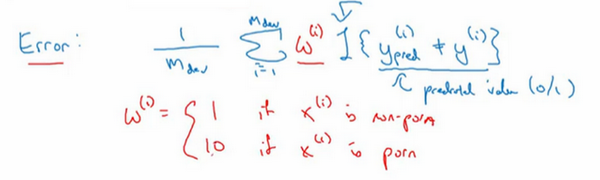$Error = \frac{1}{m_{{dev}}}\sum_{i = 1}^{m_{{dev}}}{w^{(i)}I\{ y_{{pred}}^{(i)} \neq y^{(i)}\}}$$Error = \frac{1}{\sum_{}^{}w^{(i)}}\sum_{i = 1}^{m_{{dev}}}{w^{(i)}I\{ y_{{pred}}^{(i)} \neq y^{(i)}\}}$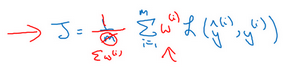$J=\frac{1}{\sum{{{w}^{(i)}}}}\sum\limits_{i=1}^{m}{{{w}^{(i)}}L({{\hat y}^{(i)}},{{y}^{(i)}})}$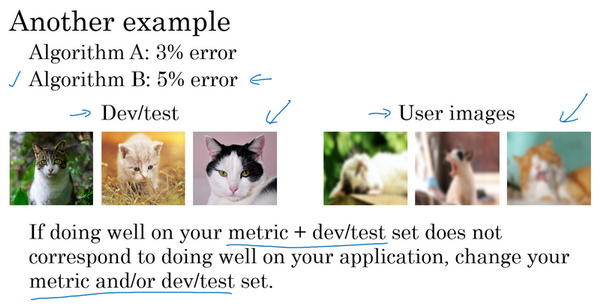### 1.8 为什么是人的表现？（Why human-level performance?）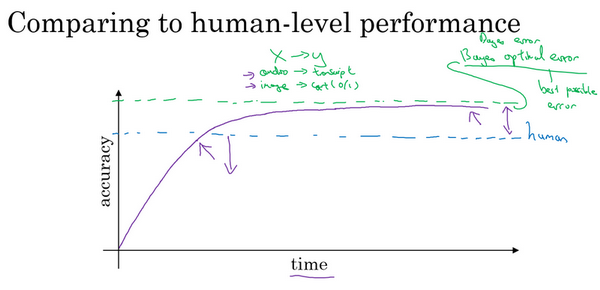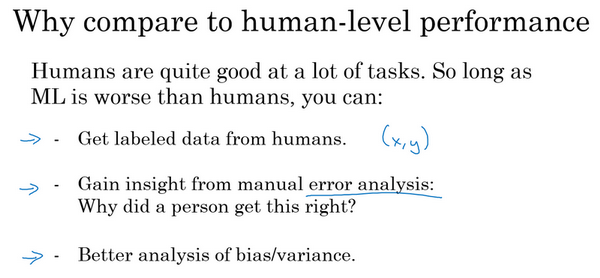### 1.9 可避免偏差（Avoidable bias）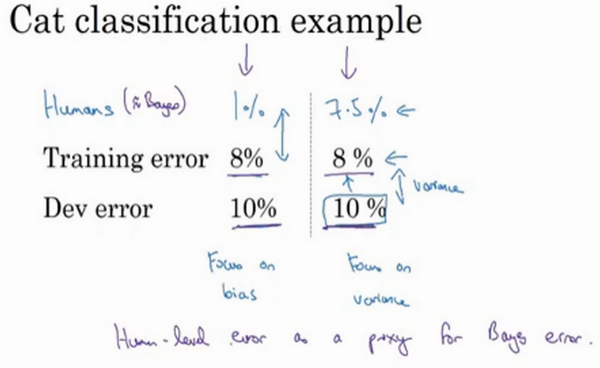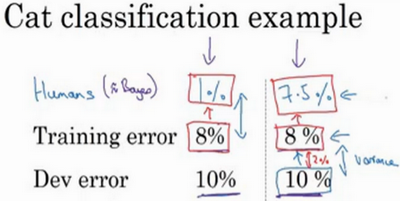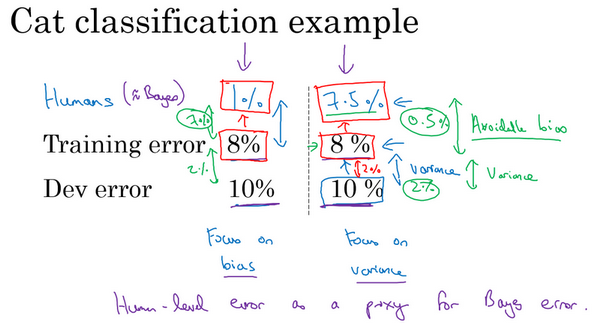### 1.10 理解人的表现（Understanding human-level performance）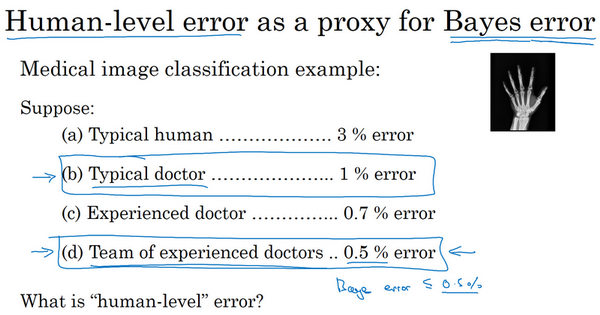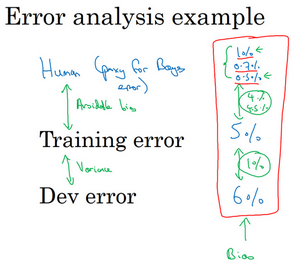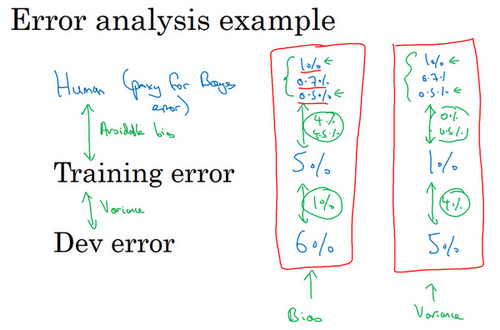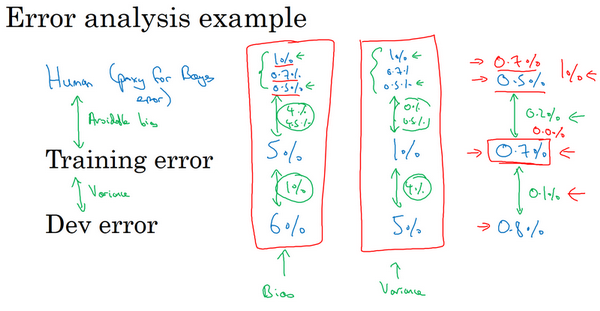### 1.11 超过人的表现（Surpassing human- level performance）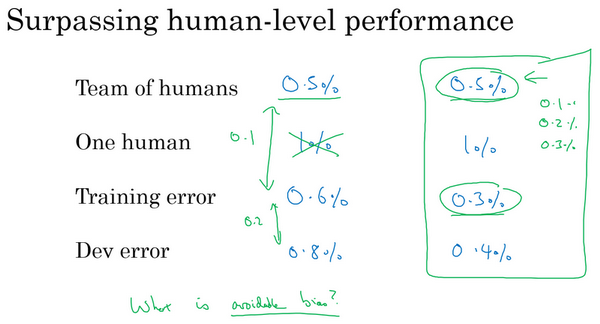### 1.12 改善你的模型的表现（Improving your model performance）